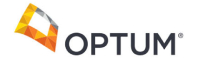New update is available. Click here to update.
Topics

Hard0/120
Average time to solve is 20m## Problem statement

You are given a non-negative integer represented as a linked list of digits (‘N’ is the number of nodes in the linked list). The digits are stored so that the head of the linked list stores the most significant digit.

Example:
``````Input: ‘N’ = 3, ‘head’ = [2, 3, 4]

Output: [2, 3, 5]

Explanation: Given number is 234. After adding 1 to it, it becomes 235.
``````
Detailed explanation ( Input/output format, Notes, Images )
Constraints:
``````1 <= T <= 10
1 <= N <= 100
0 <= Node.value <= 9
``````

The number in the linked list doesn’t contain any leading zeroes except the number 0, where there’s only one node with the value 0.

``````Time Limit: 1-sec
``````
Sample Input 1:
``````2
3
2 3 4

4
1 1 1 9
``````
Sample Output 1:
``````2 3 5
1 1 2 0
``````
Explanation Of Sample Input 1:
``````Input: ‘N’ = 3, ‘head’ = [2, 3, 4]

Output: [2, 3, 5]

Explanation: Given number is 234. After adding 1 to it, it becomes 235.

For test case 2:

Input: ‘N’ = 4, ‘head’ = [1, 1, 1, 9]

Output: [1, 1, 2, 0]

Explanation: Given number is 1119. After adding 1 to it, it becomes 1120.
``````
Sample Input 2:
``````2
4
1 9 9 9

4
9 9 9 9
``````
Sample Output 2:
``````2 0 0 0
1 0 0 0 0
``````Console